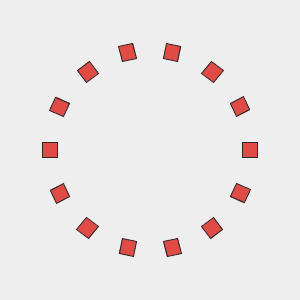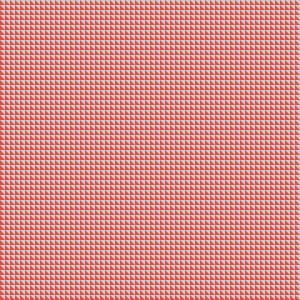for()

general description, what is it and what is it for

1. Arranging shapes around a circle

There's a simple line of code, that allows us to arrange items around a circle. The variable for the amount of items can be used to divide the circumference into a specified number of equal parts.

``````// This is a sketch template for the p5diary

color black = #222222;
color white = #eeeeee;
color red = #E14C45;

void setup() {
rectMode(CENTER);
size(300, 300);
fill(red);
background(white);
stroke(black);
float segmentCount = 14;
translate(width/2, height/2);
for (float i = 0; i < segmentCount; i++) {
rect(100, 0, 15, 15);
}
}

void draw() {
}
``````Nested for-loops

a grid of triangles

``````// This is a sketch template for the p5diary

color black = #222222;
color white = #eeeeee;
color red = #E14C45;

void setup(){
size(300,300);
fill(red);
noStroke();
for (int i = 0; i < width; i = i+5) {
for (int j = 0; j < height; j = j+5) {
pushMatrix();
translate(i,j);
triangle(0,0,0,5,5,5);
popMatrix();
}
}
}

void draw(){
gif(1,1);
}
``````